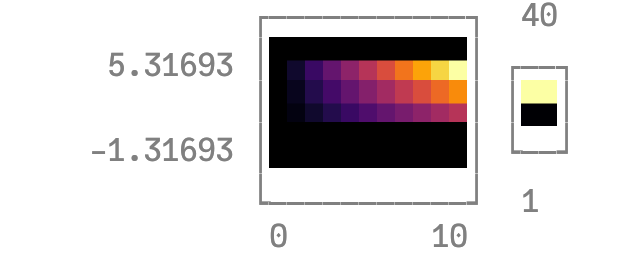# Heatmap, categorical axes, and aspect_ratiousing Plots
unicodeplots()
xs = [string("x", i) for i = 1:10]
ys = [string("y", i) for i = 1:4]
z = float((1:4) * reshape(1:10, 1, :))
heatmap(xs, ys, z, aspect_ratio = 1)